Miscellaneous

Chapter 11 Class 11 Conic Sections (Term 2)
Serial order wise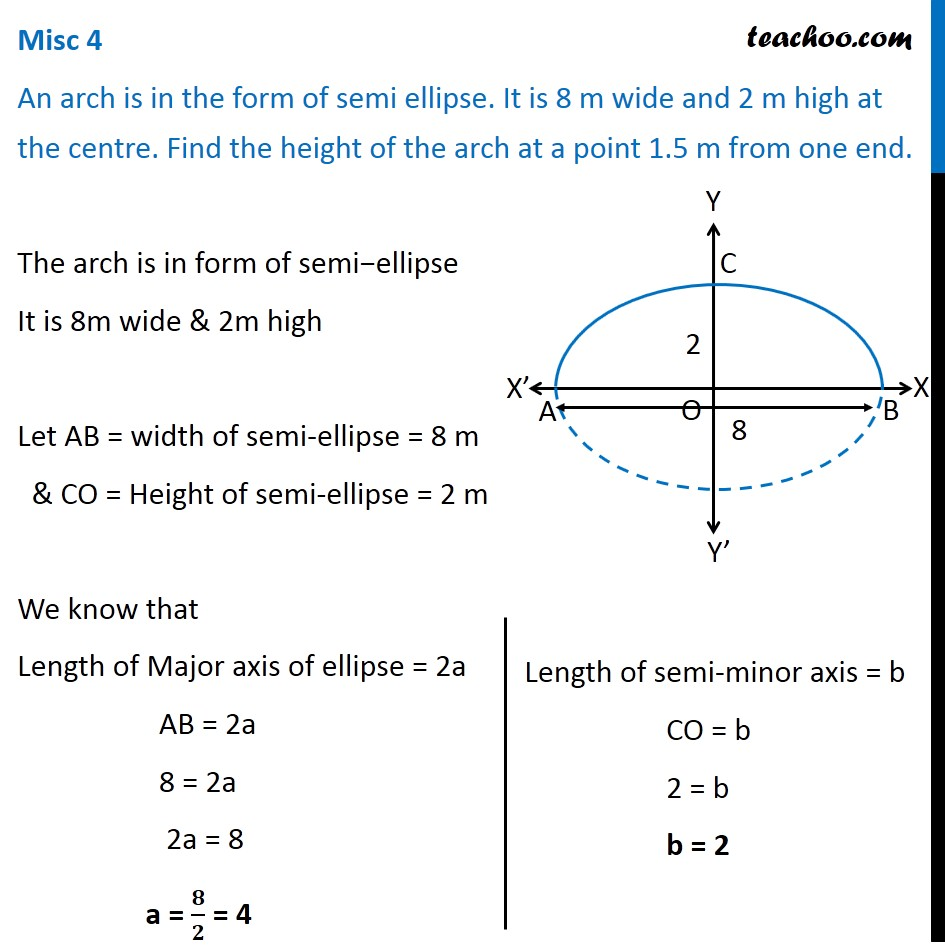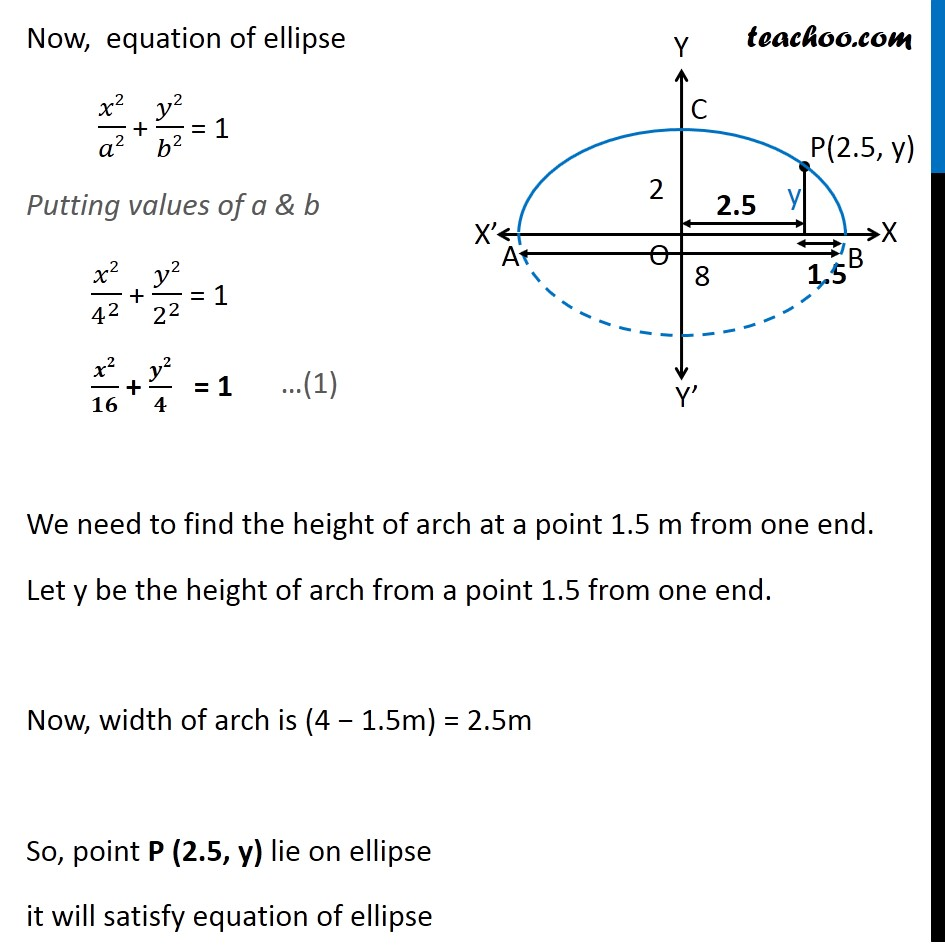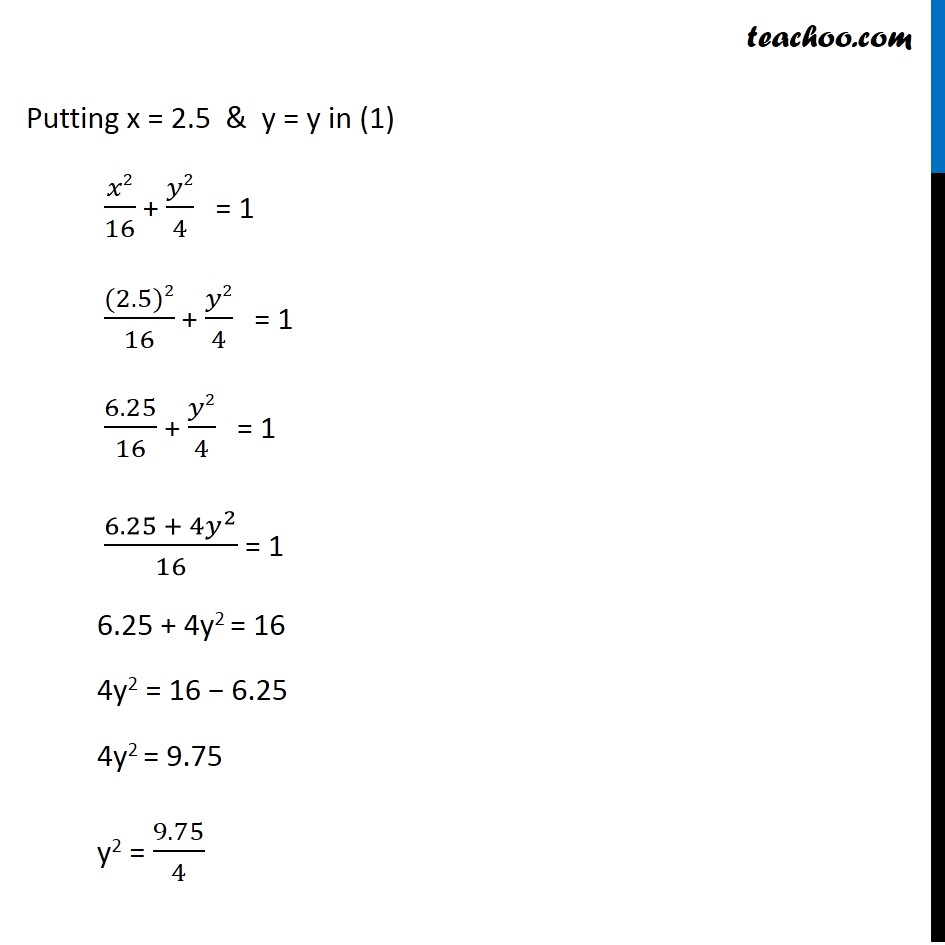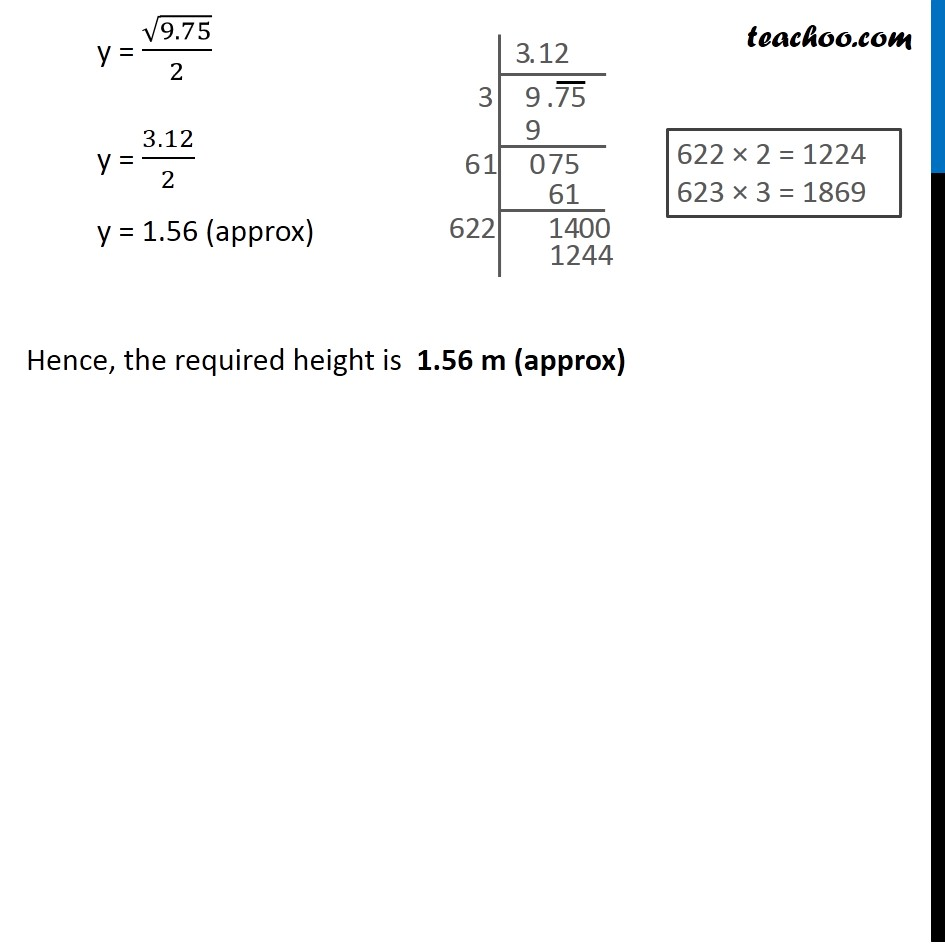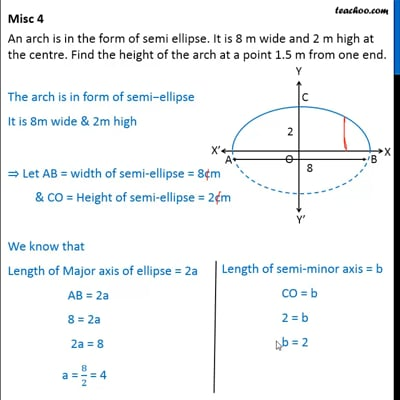This video is only available for Teachoo black users

### Transcript

Misc 4 An arch is in the form of semi ellipse. It is 8 m wide and 2 m high at the centre. Find the height of the arch at a point 1.5 m from one end. The arch is in form of semi−ellipse It is 8m wide & 2m high Let AB = width of semi-ellipse = 8 m & CO = Height of semi-ellipse = 2 m We know that Length of Major axis of ellipse = 2a AB = 2a 8 = 2a 2a = 8 a = 𝟖/𝟐 = 4 Length of semi-minor axis = b CO = b 2 = b b = 2 Now, equation of ellipse 𝑥2/𝑎2 + 𝑦2/𝑏2 = 1 Putting values of a & b 𝑥2/4^2 + 𝑦2/2^2 = 1 𝒙𝟐/𝟏𝟔 + 𝒚𝟐/𝟒 = 1 We need to find the height of arch at a point 1.5 m from one end. Let y be the height of arch from a point 1.5 from one end. Now, width of arch is (4 − 1.5m) = 2.5m So, point P (2.5, y) lie on ellipse it will satisfy equation of ellipse Putting x = 2.5 & y = y in (1) 𝑥2/16 + 𝑦2/4 = 1 ((2.5)2)/16 + 𝑦2/4 = 1 6.25/16 + 𝑦2/4 = 1 (6.25 + 4𝑦^2)/16 = 1 6.25 + 4y2 = 16 4y2 = 16 − 6.25 4y2 = 9.75 y2 = 9.75/4 y = √9.75/2 y = 3.12/2 y = 1.56 (approx) Hence, the required height is 1.56 m (approx)